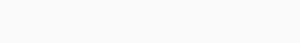#Quants Questions : Number System Set 2

Hello Aspirants. Welcome to Online Quantitative Aptitude Section with explanation in AffairsCloud.com. Here we are creating question sample in Number System, which is common for all the competitive exams. We have included Some questions that are repeatedly asked in bank exams !!!

1. Two third of a positive number and 25/216 of its reciprocal are equal.The number is
A)12/5
B)25/144
C)5/12
D)144/25
Explanation :
(2/3)x = (25/216)(1/x)
X^2 = (25×3)/(216×2) = 25/144
X = 5/12

2. If 2(1/2) is added to a number and the sum multiplied by 4(1/2)and 3 is added to the product and then divide the sum by 1(1/5), the quotient becomes 25 .What is the number ?
A)5(1/2)
B)4(1/2)
C)2(1/2)
D)3(1/2)
Explanation :
{4(1/2)[X+2(1/2)]+3}/[1(1/5)] = 25
(9X/2)+(45/4)+3 = 25×(6/5)
9X/2 =63/4
X=7/2 or 3(1/2)

3. In a division sum ,the divisor is 3 times the quotient and 6 times the remainder.If the remainder is 2 then the dividend is
A)36
B)40
C)45
D)50
Explanation :
Divident = Quotient × Divisor +Remainder
Divisor = 6×2 = 12
Q = 12/3 =4
Divident = 4×12+3 =48+2 = 50

4. The sum of two number is 24.The greatest product of these two numbers can  be
A)124
B)144
C)164
D)140
Explanation :
X + Y = 24
Greatest Product then x and y must be equal
Possibility of getting 24
12+12= 24
12×12 = 144

5. Twenty times a positive integer is less than its square by 96.What is the integer ?
A)24
B)4
C)-24
D)-4
Explanation :
X^2 – 20X = 96
X^2-20X-96 = 0
(X-24)(X+4) = 0
X= 24, -4
positive integer = 24

6. If (a-b) 3, (b-c) = 5 and (c-a) = 1 then the value of (a^3+b^3+c^3-3abc)/(a+b+c)
A)17
B)17.5
C)18
D)18.5
Explanation :7. The product of the 2 consecutive odd number is 6723.What is the greater number ?
A)80
B)81
C)82
D)83
Explanation :
X ×(X+2) = 6723
X^2 + 2X = 6723
X^2+2X-6723 = 0
(X – 81)(X+83) = 0
Greatest number = 83

8. The sum of three number is 264. If the first number be twice the second and third number be one third of the first then the second number is
A)82
B)72
C)76
D)87
Explanation :
2X+X+(2X/3) = 264
(6X+3X+2X)/3 = 264
11X/3 = 264
X= (264×3)/11 = 72

9. If the number obtained on interchanging the digits of a 2 digit number is 18 more than the original number and the sum of the digit is 8.Then what is the original number?
A)35
B)53
C)32
D)34
Explanation :
Unit digit= x ;  Ten’s digit = (8-x)
[10(8-x)+x] – [10x+(8-x)] = 18
80-10x+x-10x-8+x = 18
-18x = 18-80+8
18x = 54
X = 54/18 = 3
8 – x = 8 – 3 =5
Hence the number is 35

10. The sum of three consecutive multiple of 4 is 72 .What is the largest number ?
A)26
B)20
C)28
D)14